Market Organization and Structure | IFT World
IFT Notes for Level I CFA® Program
IFT Notes for Level I CFA® Program

# Part 4

## 10. Positions and Short Positions

An investor’s position in a security may either be a long position or a short position.

Long positions

• These are created when a trader owns an asset or has a right or obligation under a contract to purchase an asset.
• Investors who are long benefit from an increase in price of the security.
• A long position can be levered or unlevered.

Short positions

• These are created when traders borrow an asset and sell it, with the obligation to replace the asset in the future.
• Investors who are short benefit from a decrease in price of the security.

We will now look at each of these positions in detail.

### 10.1 Short Positions

Short positions are created when traders sell contracts or stocks they do not own. It is similar to borrowing an asset you do not own.

How to create a short position in a security:

Example of a short position:

Assume you research a stock – Oracle Corporation – and forecast its price to go down in the short term. The holder of a short position benefits when the security price goes down. To profit from this view, you borrow securities from a long party and sell the borrowed Oracle stock to other traders when it is trading at $50. The stock falls to$40 in line with your forecast. You then close the position by repurchasing and delivering it to the long party, profiting $10 per share in the process. The potential gain is bounded, in our example, to a maximum of$50. That is, the maximum profit you can earn is $50 if the stock falls from$50 to $0. Conversely, the potential loss is unbounded. If the stock’s price increases instead of falling, then the short seller incurs a loss and theoretically, there is no maximum limit to the loss. This makes a short position very risky. For a long position, the reverse happens. If you own Oracle stock and the stock’s price increases, there is no limit to the maximum profit you can make. However, the loss if the stock falls is limited to$50.

To secure the security loans given to short sellers, security lenders require that proceeds of the short sale be posted as collateral ($50 in the example above). The security lender then invests the proceeds in short term securities and pays interest on collateral to short sellers at rates known as short rebate rates. Security lenders lend their securities because the short rebate rates they pay on the collateral are lower than the interest rates they receive from investing the collateral. Short Position: sell the stock (owe the asset) Maximum gain = 100 % of investment Maximum loss = unbounded Long Position: buy the stock (own the asset) Maximum gain = unlimited Maximum loss = 100% of investment ## 11. Leveraged Positions In some markets, traders are allowed to buy securities by borrowing some percentage of the purchase price. The leverage ratio is a measure of the amount borrowed relative to the total value of the asset. It shows how many times larger a position is than the equity that supports it.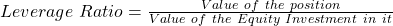The borrowed money is called the margin loan and the interest paid is called the call money rate. Traders who buy securities on margin are subject to margin requirements. The initial margin requirement is the minimum percentage of the purchase price that must be paid by the trader (called trader’s equity). Traders usually borrow money from their brokers. The advantage of buying securities on margin is that it increases the amount of profit a trader makes if the share price goes up. If the share price falls to a certain level (the margin call price) the trader will receive a call from the broker (lender) and will be asked to add more money to his account. The minimum amount of equity to be maintained in the positions is called the maintenance margin requirement. Traders receive a margin call when equity falls below the maintenance margin requirement.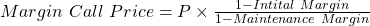Example Your broker allows you to purchase stocks on margin. The initial margin requirement is 40% and the maintenance margin requirement is 25%. You purchase a stock for$50 using $20 of your money and you borrow the rest from the broker. The interest rate on borrowed money is 5%. What is the leverage ratio? At what rate will you receive a margin call? Solution: You borrow$30, your equity is $20 and the total value of the asset is$50.

The leverage ratio is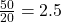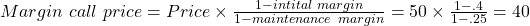If the stock price comes down to 40, you still owe the $30 and your equity has come down to$10. This is 25% of $40 (the asset price). If the stock price falls below$40 the equity becomes less than 25%, the maintenance margin. In this situation, the broker (lender) will ask you to add money to your account such that your equity is at least 25%.

Example

We continue with the earlier example where your initial margin requirement is 40%. You believe stock X will go down in price and decide to short sell 500 shares at the current price of $30. How does the margin requirement impact you? Solution: Proceeds from short sale = 500 *$30 = $15,000. Just like long buyers buy on margin, even short sellers are required to post a margin amount as a security. If the price goes up, then it is a loss for the short seller (you); to mitigate this risk of loss, the broker requires margin traders to maintain a minimum amount of equity in their positions called the maintenance margin requirement. The margin amount required here is 0.4 * 15,000 =$6,000.

The total return to the equity investment in a levered position considers:

Profit or loss on the position
–   Margin interest paid
–   Sales commission
To calculate the return percentage on a leveraged position, we need to divide the total profit by the initial investment. This is illustrated below:

Example

What is the overall return in percentage terms given the following data?

Purchase price = 30

Sales price = 32

Shares purchased = 500

Leverage ratio = 2

Call money rate = 5%

Dividend = $0.50 per share Commission =$0.02 per share

Solution: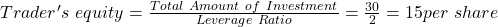i.e the remaining 15 is borrowed.

Initial investment: (Equity + Commission) x (Number of shares purchased) = 15.02 x 500 = 7,510

Trader’s remaining equity after the sale can be computed as:

Proceeds from the sale:           16,000 (32 x 500)

Payoff loan                                  -7,500 (15 x 500)

Margin interest paid                 -375 (0.05 x 15 x 500)

Dividends received                     250 (0.5 x 500)

Sales commission paid              -10 (0.02 x 500)

Remaining equity                         8365

Total profit = equity at end – initial investment = 8,365 – 7,510 = 855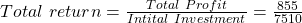= 11.38%

Crash Courses for November CFA Level I, II exams are coming soon!
This is default text for notification bar